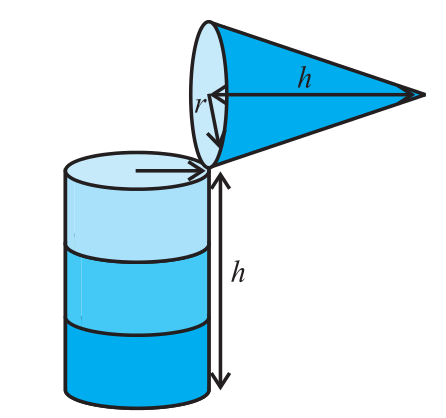Volume of right circular cone

## Objective:

To give a suggestive demonstration of the formula for the volume of a right circular cone.

## Related terms

### Cone:A cone is a three-dimensional geometric shape that tapers smoothly from a flat base (usually circular) to a point called the apex or vertex. More precisely, it is the solid figure bounded by a base in a plane and by a surface (called the lateral surface) formed by the locus of all straight line segments joining the apex to the perimeter of the base, such that there is a circular cross section. The term "cone" sometimes refers just to the surface of this solid figure, or just to the lateral surface. The axis of a cone is the straight line (if any), passing through the apex, about which the base has a rotational symmetry. In common usage in elementary geometry, cones are assumed to be right circular, where right means that the axis passes through the centre of the base (suitably defined) at right angles to its plane, and circular means that the base is a circle. Contrasted with right cones are oblique cones, in which the axis does not pass perpendicularly through the centre of the base.In general, however, the base may be any shape that permits a circular cross section of the cone, and the apex may lie anywhere (though it is usually assumed that the base is bounded and therefore has finite area, and that the apex lies outside the plane of the base). The more general conic solid also has an apex and lines connecting the apex to all the points on a planar base, which can be of any shape. If the result has a circular cross section, it is a cone; if it has a polygonal base, it is a pyramid.

## Volume:Given the radius 'r' and height 'h', the volume of a cone can be found by using the formula:

Formula: Vol. cone = 1/3 × b × h.  b is the area of the base of the cone. Since the base is a circle, area of the base = π × r2

Thus, the formula is Vol. cone = 1/3 × π × r2 × h  Use π = 3.14